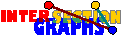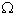# Intersection Classes

One might think that intersection graphs are very special graphs, but this is not the case. Actually every graph G=(V,E) is an intersection graph [M45]. The construction is very simple. For every vertex xV we define Sx as the set of those edges containing x. Obviously two distinct vertices are adjacent iff they lie in some common edge, that is, iff SxSy is nonempty. This result also shows that this 0-1 optimization problem is NP-complete.

But in the applications, usually one is interested in intersection representations of a certain type only. In the most general situation we have some hypergraph property H and are interested in intersection graphs of members of H. We will treat below the most tamest classes. If the class H is at least closed under subhypergraphs, then the intersection class is closed under induced subgraphs. Intersection graphs of linear k-uniform hypergraphs are an example of such a class (although the class is no Scheinerman class).

The classes of

are examples of tougher classes, where not even that is true.

### Scheinerman classes

The simplest possible restrictions are restrictions on the sets Sx. That is, we have some set property P and are interested in intersection graphs of hypergraphs where all sets Sx have to obey property P. We will even require that the all sets obeying property P form a set which we will denote by P too. The class of all such finite (!) intersection graphs will be denoted by G(P).

Sometimes we do not allow multiple sets, i.e. we require the hypergraph to be simple. The class of intersection graphs of simple hypergraphs where all hyperedges obey property P is denoted by G0(P).

Classes of the form G(P) or G0(P) are called a Scheinerman classes, see a table of examples. Mostly the two concepts do not make much difference, at least as far as recognition is concerned. Actually it can be proved that recognizing members of G(P) cannot be more difficult than recognizing members of G0(P). To explain this connection between the classes, we have to define the reduct R(G) of a graph G as follows: Two vertices x and y are equivalent if they have the same closed neighborhood. This is an equivalence relation, admissible with the adjacency relation, i.e. if x, y are equivalent, and if z, v are equivalent, then x and z are adjacent if and only if y and v are. Therefore the quotient graph R(G) can be defined, which has the equivalence classes as vertices, and two such classes adjacent iff any two vertices of the corresponding classes are adjacent in G.A graph G lies in G(P) if and only if its reduct R(G) lies in G0(P)
 Proof: Note that we can view the reduct to be a subgraph induced by a complete system W of representants of the equivalence classes. Assume G=((A,Sx/xV))), where all Sx obey P. Then R(G) =( (A,Sw/ wW))). If Sw and Su were equal, for distinct u,wW, they would have to be equivalent in R(G), and in G also, a contradiction. Conversely, let R(G)=((A,Sw/ wW))), with all Sw distinct and obeying P. For every xV \ W there is some wW equivalent to x in G. We define Sx := Sw and get a representation of G by P-sets in this way.

Note that R(G) can be computed in polynomial time.

Since in general, we can slightly change the sets of a geometric intersection representation without affecting its intersection graph, the distinction between G(P) or G0(P) is only important for discrete intersection models.

Surprisingly, if we are only interested in finite graphs, we may require that P is countable:[S85] For every Scheinerman class G(P) there is a countable subset P´ of P such that G(P)= G(P´).
 Proof: Since for every integer n there are only finitely many graphs with n vertices, G(P) is countable, say {G1, G2,...}. For every Gi we choose a fixed representation by P-sets. For every such i, only finitely many P-sets are needed, therefore the set P´ of all those P-sets used in this way is countable.

Scheinerman classes have certain properties:

(1) If G is a member of the class, then every induced subgraph must also be a member of the class.

Even a stronger property holds:

(1´) There is some countable graph U such that the members of the class are just the finite induced subgraphs of U.

The third property holds only for classes of the type G(P):

(2) If R(G) = R(F) and if F lies in G(P), then G also.

Interestingly, these two or three properties characterize Scheinerman classes:[S85]: For a graph class G, there is some property P such that G = G(P) (respectively G=G0(P)) if and only if (1´) and (2) above (respectively (1´)) hold.
 Proof: Let x(1),x(2),... be the vertices of U=(V,E) (of condition (1´)). We define Sx(1),Sx(2),... one after another, taking care at every step that Sx(i) intersects all Sx(j), j < i, x(i)x(j)E, but no Sx(j), j < i, where x(i)x(j) does not lie in E. This can be achieved at every step provided the `private part' of every Sx(j)---those elements that do not lie in any other Sx(t) chosen so far---remains infinite at any step.

Since several graph classes obey these properties above, they have characterizations by intersection models. Examples are the classes of distance-hereditary graphs, or asteroidal triple free graphs, which are both Scheinerman classes. However, being far from natural, the models constructed in the proof of Theorem 3.2 are not very useful---in most cases they do not ressemble the structure of the graphs.For which of the classes of strongly chordal graphs, distance-hereditary graphs,... is there some natural set property P such that G(P) equals the class of graphs?

An example of such a Scheinerman class, where the intersection model ---which turned out to be very useful--- was discovered only 16 years after the introduction of the class are the so-called chordal graphs. A graph is chordal if it contains no cycles of length 4 or more as induced subgraphs [G80].[B74] [G74]: Let A be the vertex set of some infinite binary tree T. A finite subset S of A has property P if it induces a subtree of T. Then G(P) equals the class of chordal graphs.

But why are the three classes mentioned Scheinerman classes? Property (1) is easy to check. For (1´), note that in these three examples the disjoint union of two graphs of the class lies again in the class. Then we can define U as the disjoint union of all (finite) graphs in the class.

Back to the start page for intersection graphs.

Erich Prisner